# Add numbers with up to 4 digits together

## Learning focus

Learn how to add numbers with up to 4 digits together.

This includes:

• a catch-up quiz
• one video
• three slideshows

# Quiz

To get started, let's see how well you know this topic already. Take the catch-up quiz below to find out.

# Learn

Let's recap the basic method for column addition with this video from KS2 Maths.

## Column addition

The column method involves writing one number underneath the other.

Add the numbers, starting from the right.

## Example 1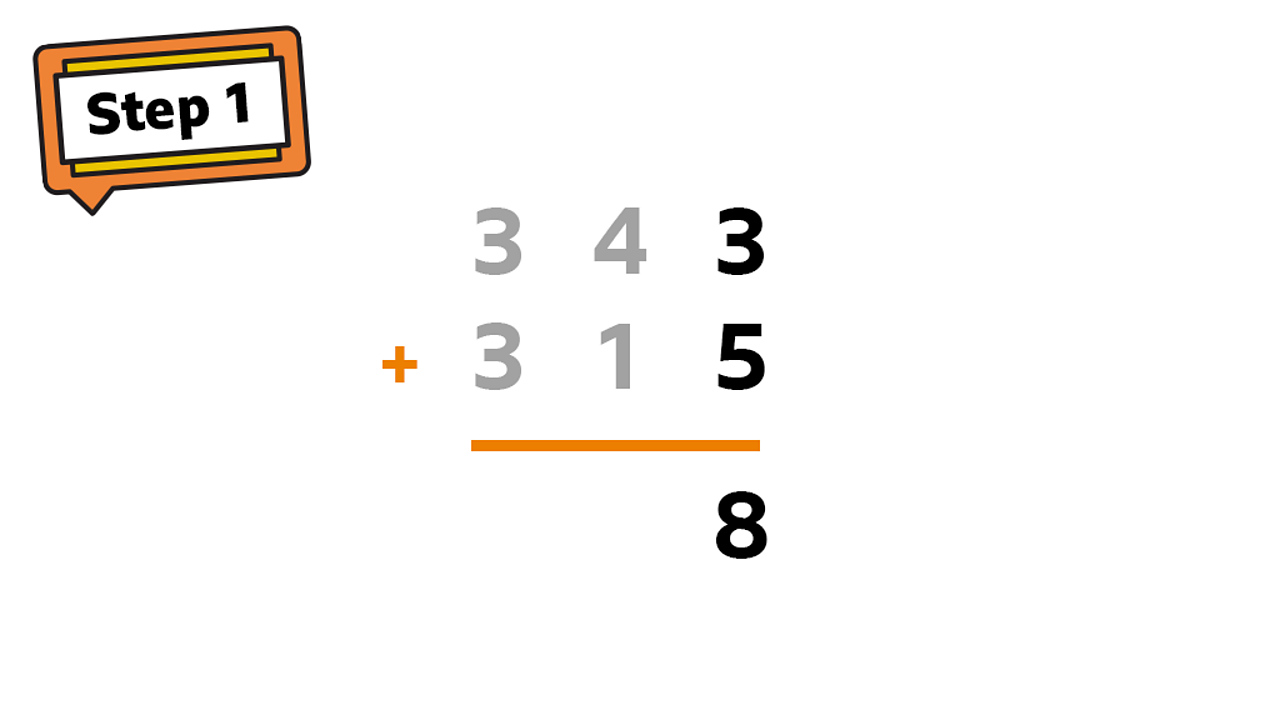Start from the ones column: 3 + 5 = 8.1 of 4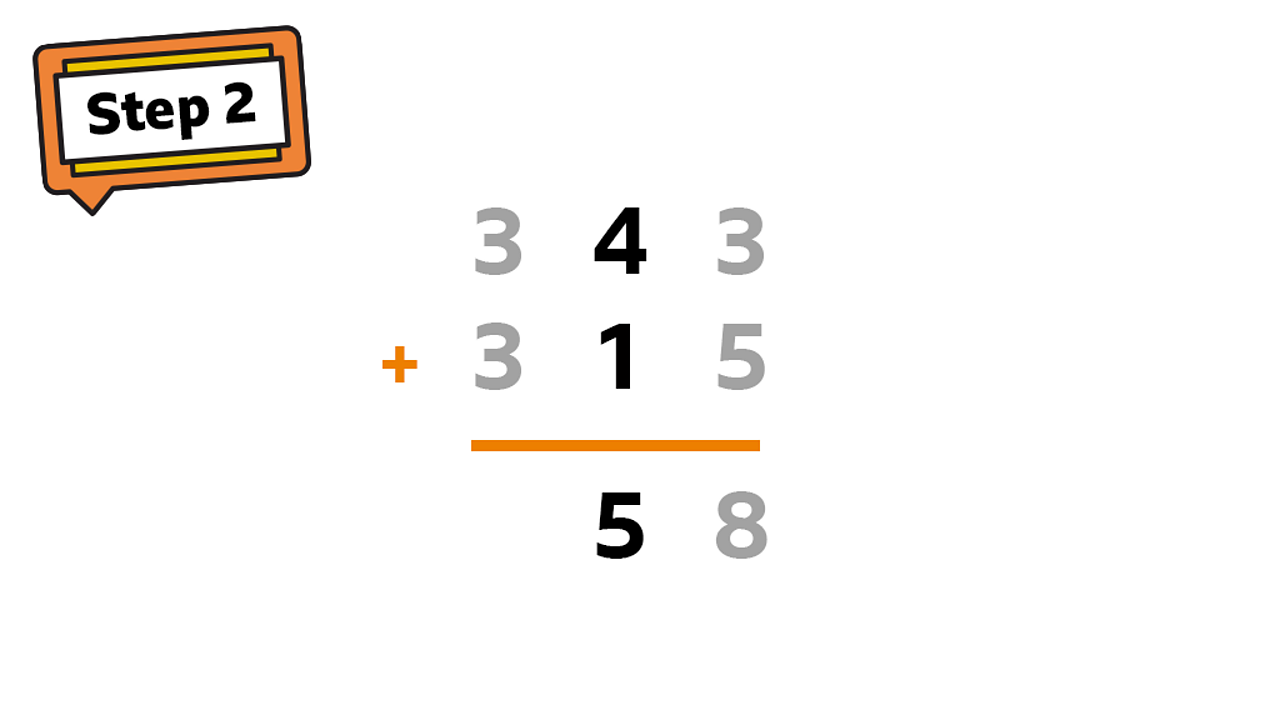In the tens column: 40 + 10 = 50.2 of 4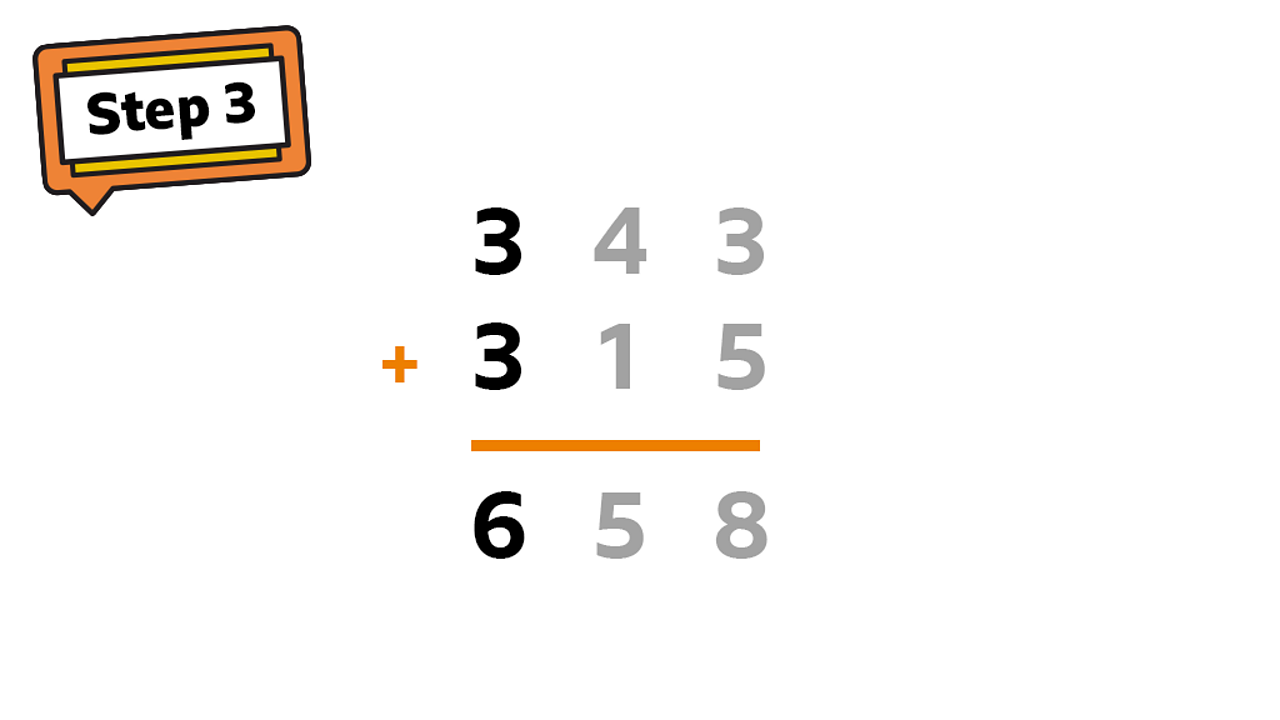In the hundreds column: 300 + 300 = 600.3 of 4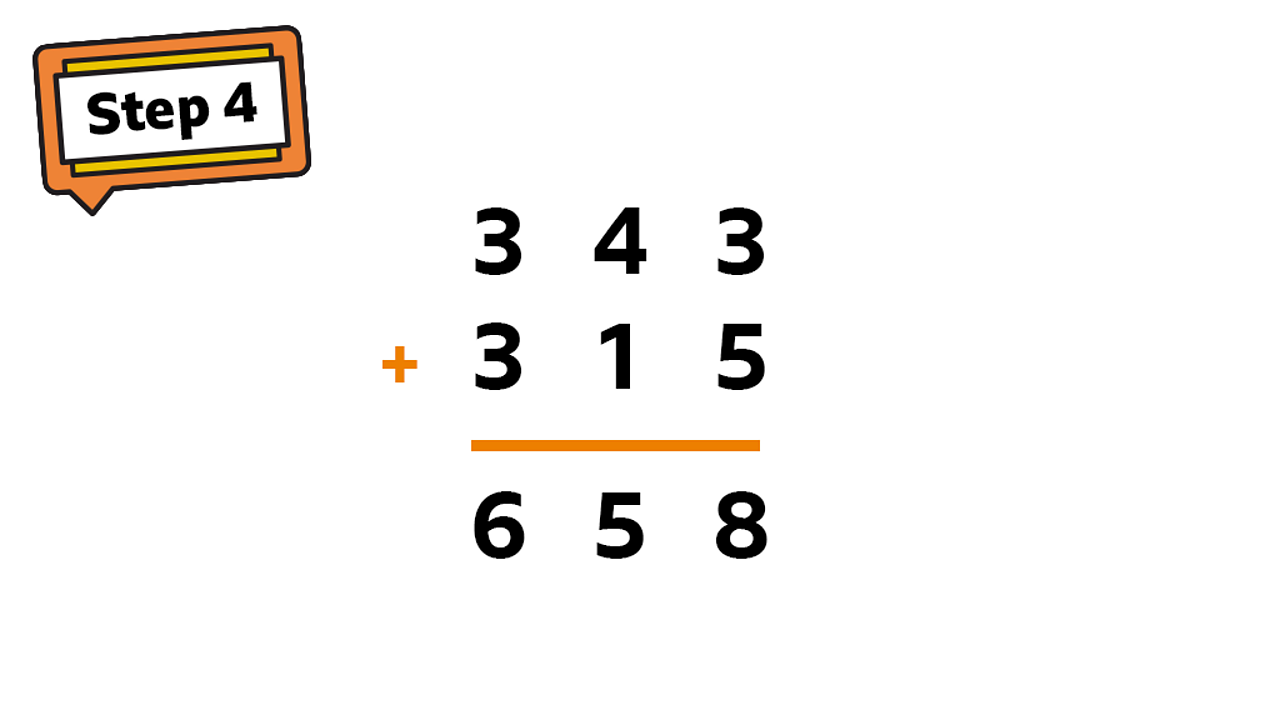The finished sum. 343 + 315 = 658.4 of 4

## Example 2

If the two numbers in a place value column add up to a number greater than 9, you must carry a digit.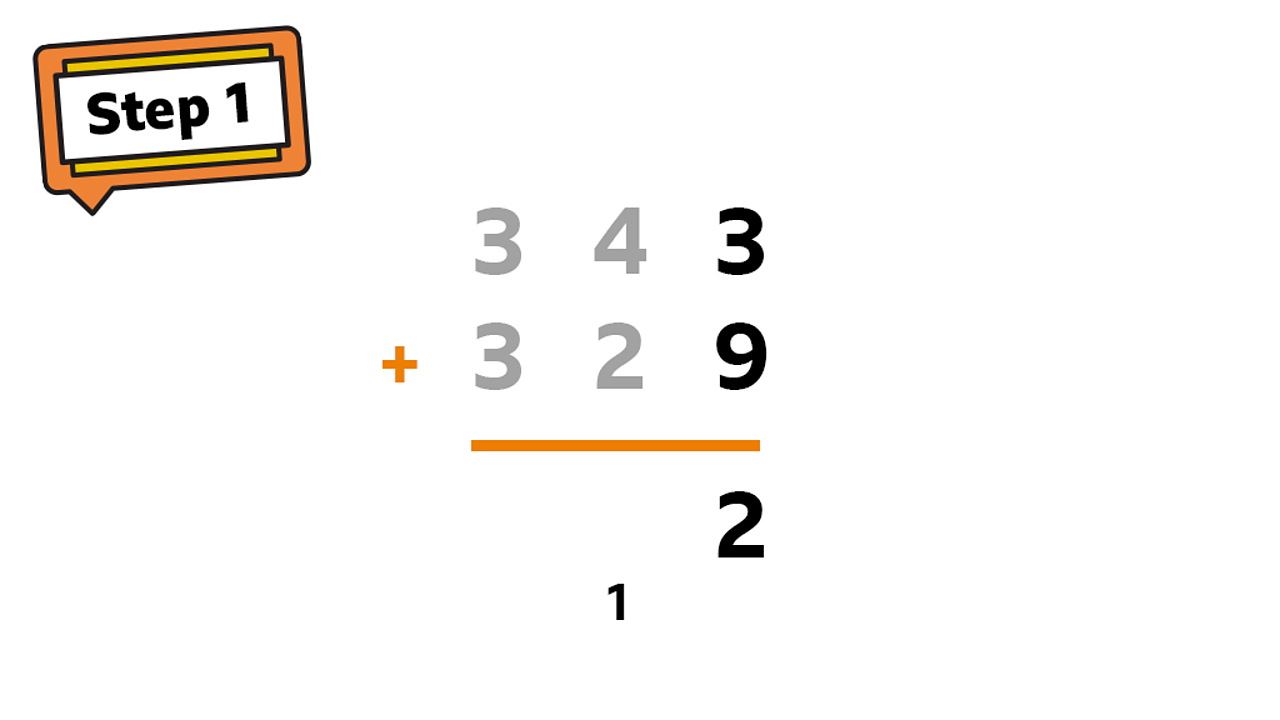Start from the ones column: 3 + 9 = 12. Write a 2 in the ones column and carry 10 to the tens column by writing a 1 below.1 of 4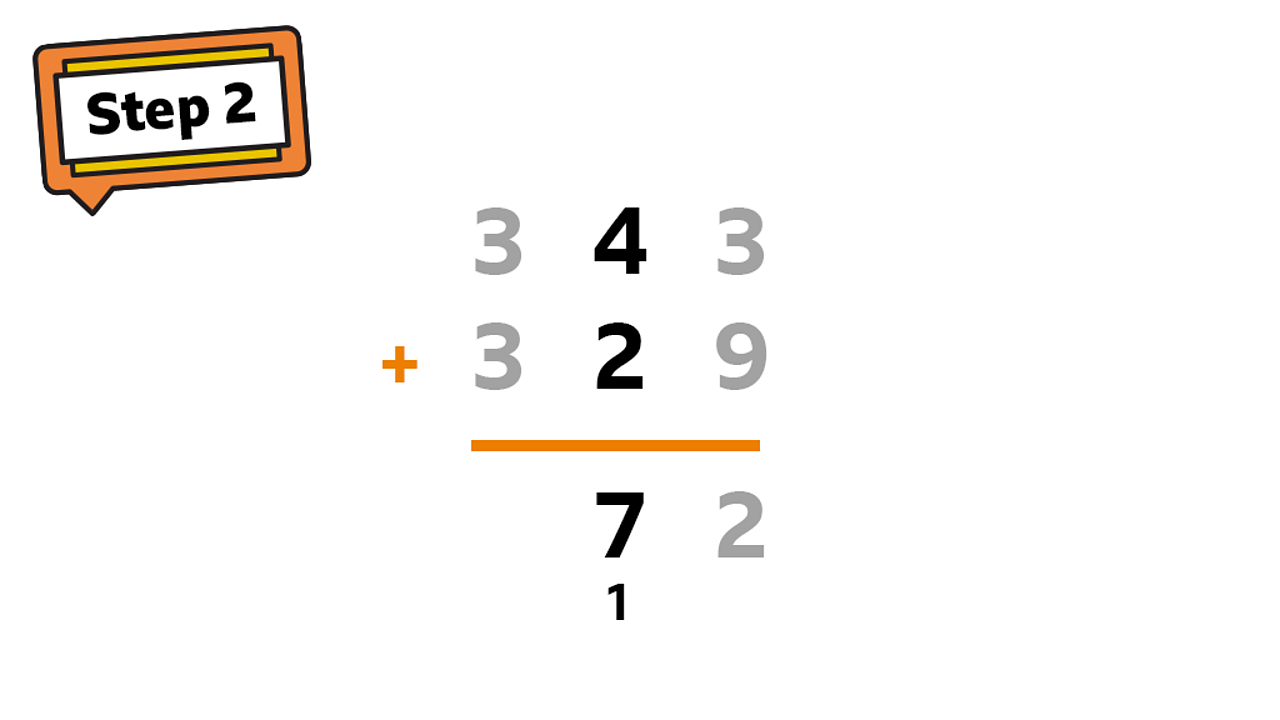In the tens column: 40 + 20 + 10 = 70. Remember to add that extra ten you carried over.2 of 4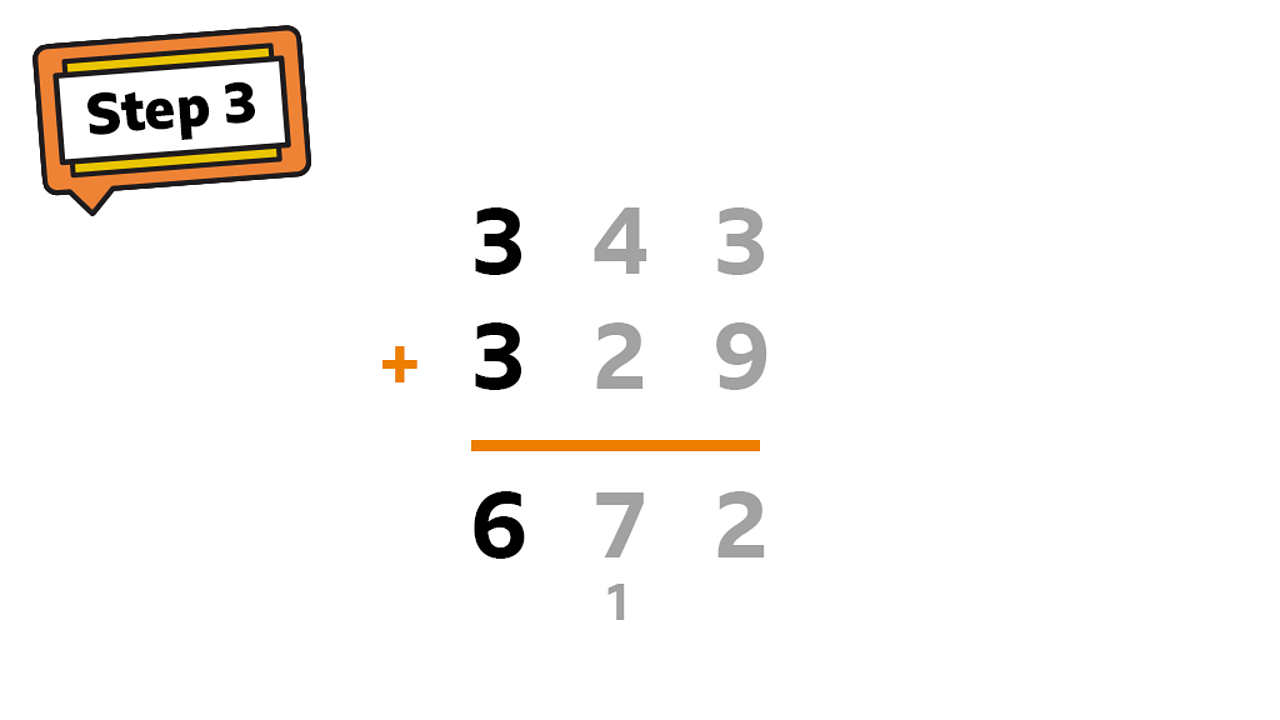In the hundreds column: 300 + 300 = 600.3 of 4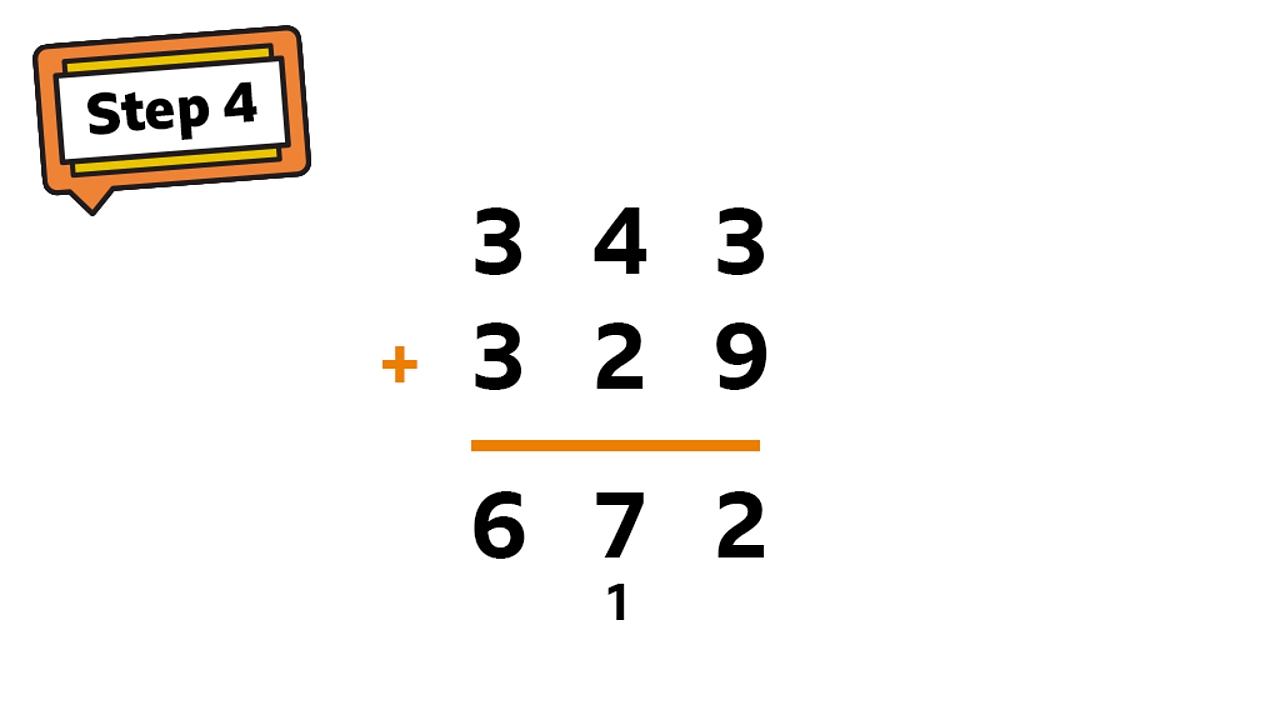The finished sum. 343 + 329 = 672.4 of 4

If you can add two 3-digit numbers, then you can use the exact same method to add 4-digit numbers together!

Just remember to add on the number that you carry over.

## What is 4214 + 3936?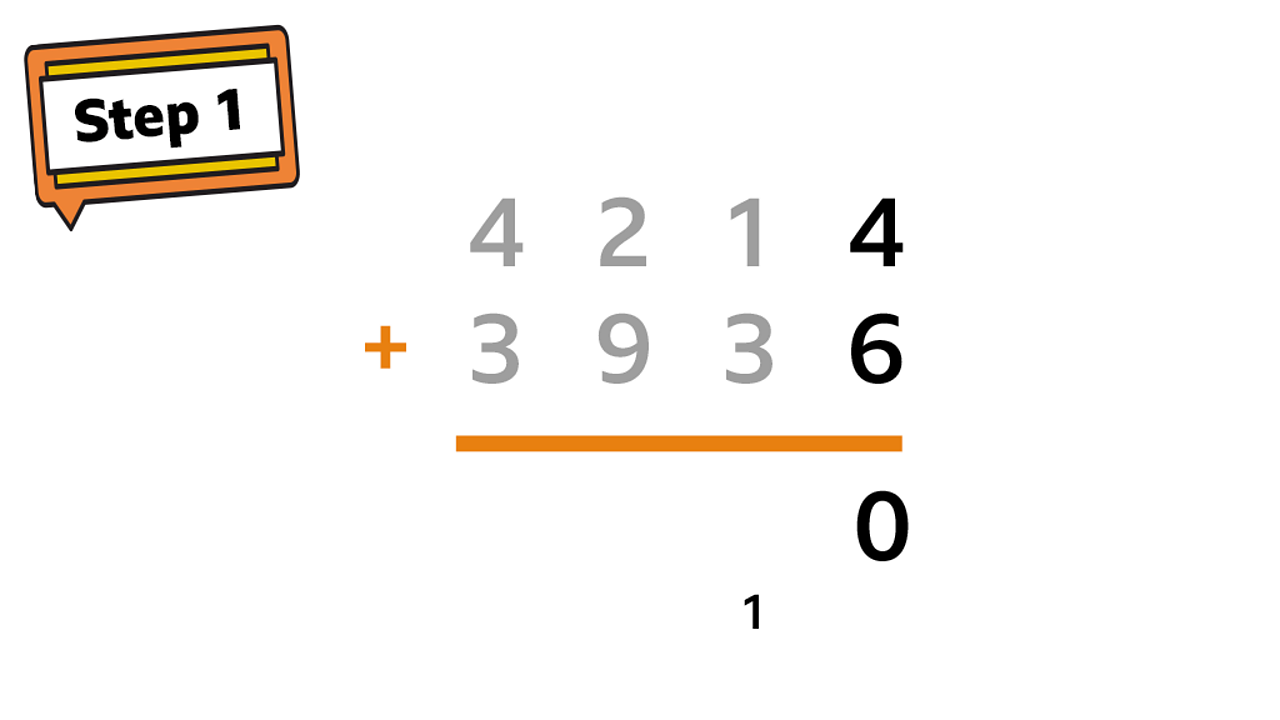As always, start by adding the ones column. 4 + 6 = 10. Place a 0 in the ones column and carry 10 over to the tens column.1 of 5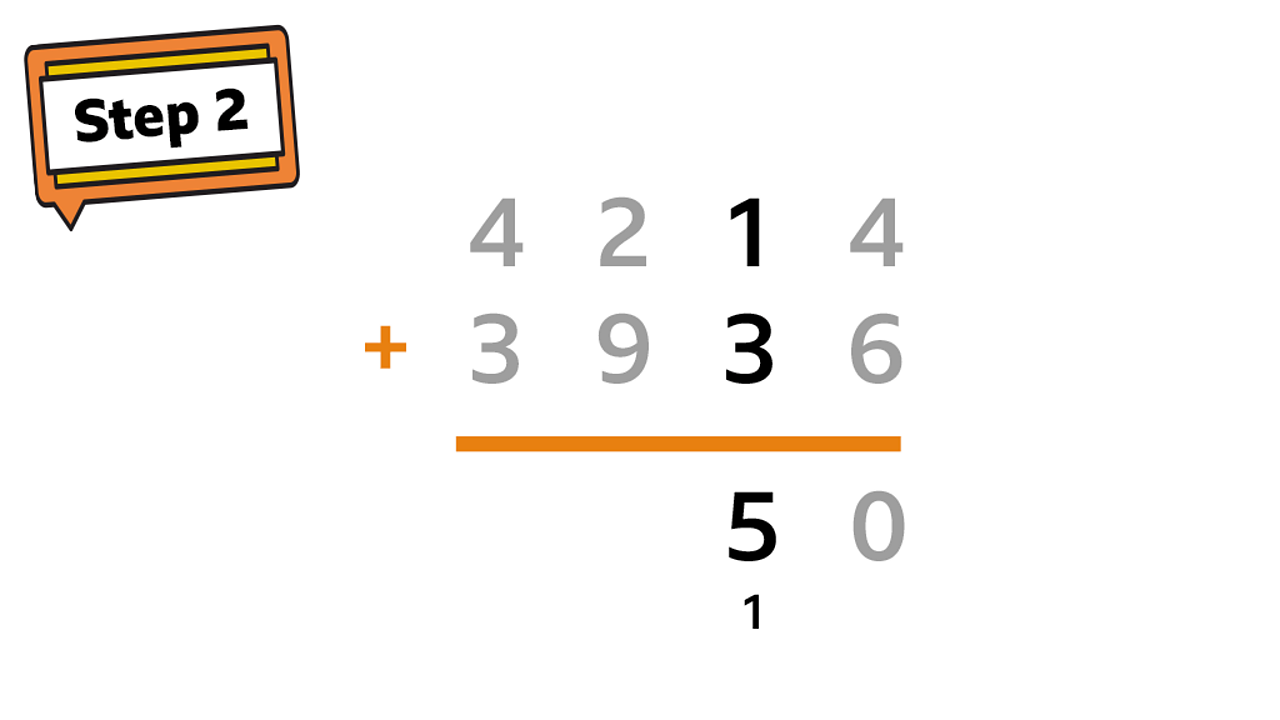Add up all the digits in the tens column. Don’t forget about the 10 that was carried over!2 of 5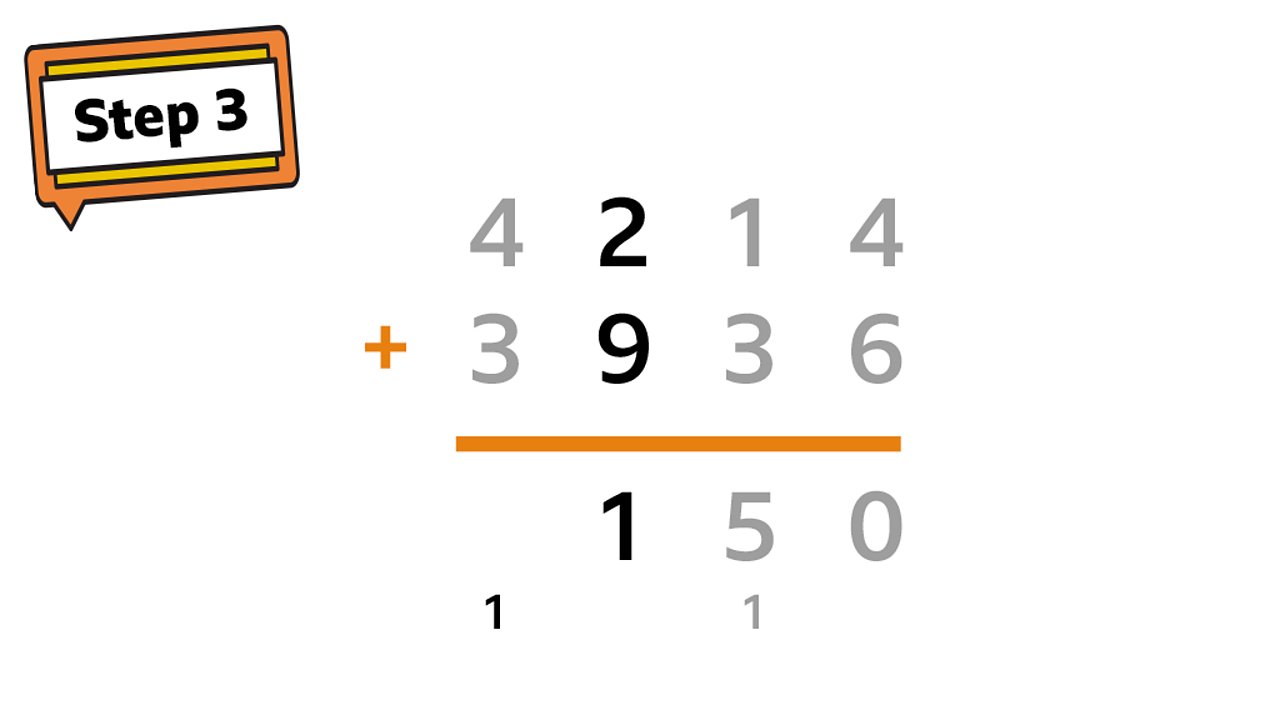Move on to the hundreds column. 200 + 900 = 1100. Put the 1 in the hundreds column and carry the 1 in the thousands over.3 of 5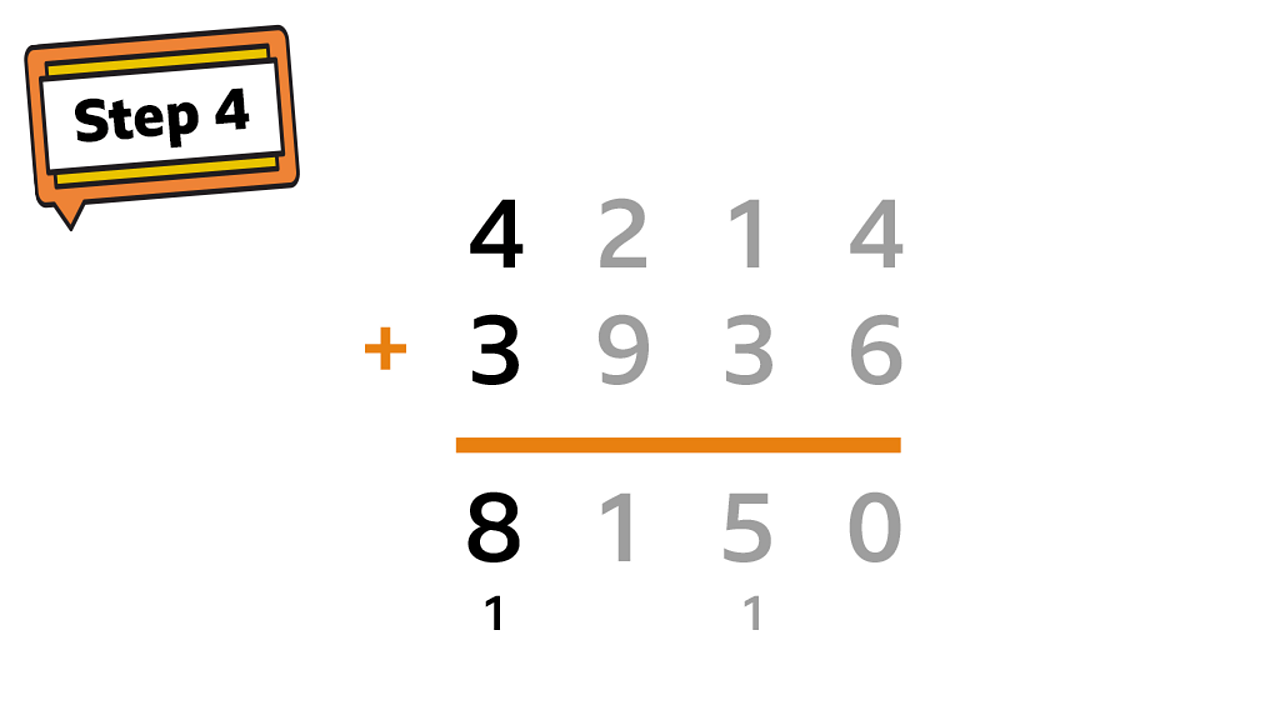Add up all the thousands to find the answer! 4000 + 3000 + 1000 = 8000.4 of 5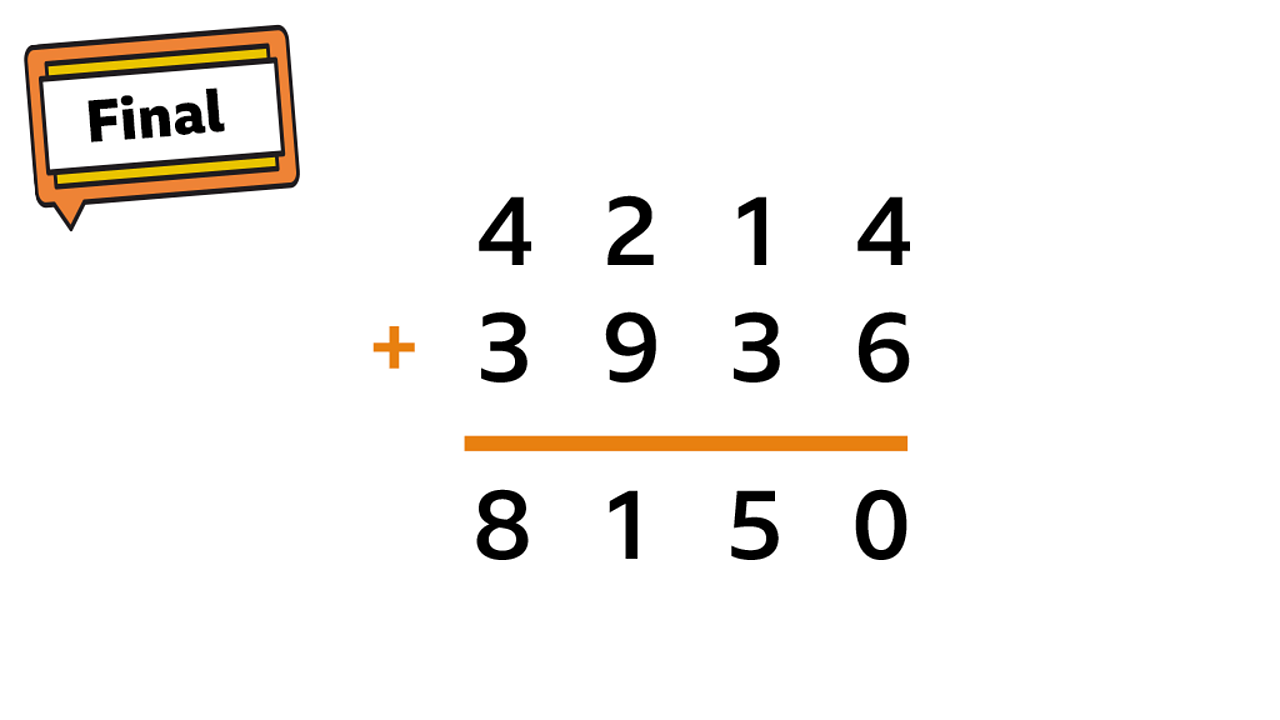4214 + 3936 = 8150.5 of 5

## Checklist

• When writing down sums, separate the numbers into ones, tens, hundreds and thousands.
• List the numbers in a column and always start adding the ones first.
• Estimate first and check afterwards - it's a good idea to estimate a rough answer and then check your actual answer.
• Order doesn't matter - for example, remember that 345 + 129 is the same as 129 + 345.
• Key words - look out for these words in problems: total, sum, altogether, more. They all indicate an addition calculation.

# Play

Play Guardians: Defenders of Mathematica to learn more and sharpen your skills on this topic.

# There's more to learn

Have a look at these other resources.